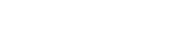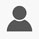Get 15%OFF On All Courses. Apply Code EDUSITY15
Price \$201.88
Enroll Now

#### Approx 26 Hours To Complete Explore Quantum Physics in English Language

This course will help you gain a greater understanding of the world, and will help you prepare for more advanced Quantum Physics courses. This course has math and teaches you important conceptual concepts.

• Quantum mechanics
• Quantum gravity
• Heisenberg Uncertainty principle
• Wave/particle duality
• Superposition
• Quantum fluctuations
• Quantum Leap
• The Thermodynamic Arrow of Time
• How to calculate a photon's energy and Photoelectric Effect
• Quantum Theory and Relativity

#### Requirements

Students should be strong in algebra and have basic knowledge of trigonometry.

#### FAQ

Q. Do I need to strong in mathematics to understand quantum physics?

A. Yes, you need a little bit of knowledge in algebra.

Q. What do you learn in quantum physics?

A. First, learn basic physics like Newtonian mechanics, electromagnetism, etc., because they are necessary for learning quantum mechanics, then learn some mathematics too.

Q. Why is quantum physics important?

A. Quantum mechanics is essential for understanding the behavior of systems at atomic length scales and smaller.

#### What you will learn

• Mathematical Formulation Of Quantum Mechanics

• Quantum States

• Dirac Equation

• Interpretations Of Quantum Mechanics

• Quantum Field Theory

• Quantum Gravity

• String Theory

• Schrodinger Equation

• What Is Quantum Physics In Simple Terms?

• What Are The Laws Of Quantum Physics?

• What Did Einstein Say About Quantum Physics?

• What Is Quantum Physics Used For?

• Particle In A One-dimensional Lattice (periodic Potential)

• Angular Momentum

• Noether's Theorem

• Pauli Exclusion Principle

• Bell's Theorem

• Bohm Interpretation

• Schwinger's Quantum Action Principle• 0 Instructor Rating
• 0 Reviews
• 0 Students
• 8 Courses

• Stars 5
• %0

• Stars 4
• %0

• Stars 3
• %0

• Stars 2
• %0

• Stars 1
• %0

## 0

Course Rating

Price \$201.88
Enroll Now
Get 15% OFF Use Code EDUSITY15

##### Includes
Do You have a Question

#### Course Features

• Lectures 19
• Quizzes 0
• Duration 26 hours
• Skill level 2
• Language English
• Assessments Yes

#### Skills You Will Acquire

• Physics
• PHYSICS CONTENT FOR THE SCIENCE STUDENTS.